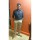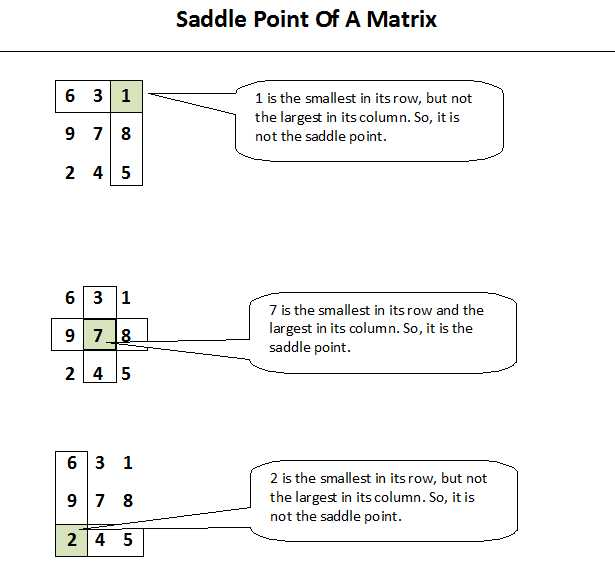Java Developers
Group for Java developers and programmers. Ask questions and get answers related to Java programming
254 Members
Join this group to post and comment.DHIRAJ LOTLIKAR
Information Technology
11 Feb 2019

# How to find Saddle point of a matrix in java?

Saddle point of a matrix is an element in the matrix which is smallest in its row and largest in its column. A matrix can have many or no saddle points. For example,

6    3     1
9    7     8
2    4    5

In this matrix, 7 is the saddle point. Because it is the smallest in its row (2nd row) and largest in its column (2nd column).### Algorithm To Find Saddle Point Of A Matrix In Java

Step 1 : For each row of the matrix, find the smallest element in a row and store it in rowMin and also store its column index in colIndex.

for (int i = 0; i < matrix.length; i++)
{
int rowMin = matrix[i];
int colIndex = 0;

for (int j = 1; j < matrix[i].length; j++)
{
if(matrix[i][j] < rowMin)
{
rowMin = matrix[i][j];
colIndex = j;
}
}
}

Step 2 : Check whether rowMin is also the largest element in its column i.e colIndex.

for (int j = 0; j < matrix.length; j++)
{
if(matrix[j][colIndex] > rowMin)
{